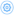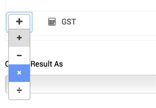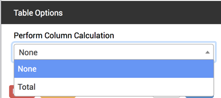# # Calculations

FormTab forms now support Calculation field types that allow you to perform mathematical operations live within your forms.

Calculations can be performed on any number or date field, making them perfect for:

• Quote/Estimate forms
• Timesheets
• Purchase orders
• And more

You can also add calculations to table rows and/or columns and perform calculations on calculations, which means you can build up complex formulae based on the values in your form and/or values you specify.

## # Sum Calculations

Sum Calculations provide a simple sum of the field values or fixed values you specify.

To add a Sum Calculation click or drag a Sum field to your form then clickto open the settings panel.• Choose Select Field to select a field from your form

The result can be displayed as:

• Whole number, rounded (123)
• Decimal (123.45)
• Currency (\$123.45)

## # Arithmetic Calculations

Arithmetic Calculations allow you to perform simple mathematical operations using the field values or fixed values you specify.

To add an Arithmetic Calculation click or drag an Arithmetic field to your form then clickto open the settings panel.• Choose Select Field to select a field from your form• Use the Operator dropdown to select addition (+), subtraction (−), multiplication (×) or division (÷) for your arithmetic calculation. You can build up a calculation using as many fields as you like.

If you are using multiple values and operators, please be aware that calculations are performed using standard BEDMASopen in new window rules.

The result can be displayed as:

• Whole number, rounded (123)
• Decimal (123.45)
• Currency (\$123.45)

## # Timeframe Calculations

Timeframe calculations allow you to calculate and display the difference between two date/time fields.

To add a Timeframe Calculation click or drag a Timeframe field to your form then click Field Settings button to open the settings panel.• Select the fields or enter the values you wish to calculate
• Use the Timeframe date type toggle toggle to switch between field selection, entering a date or using a relative date (e.g. Now).

The result can be displayed as:

• Hours:Minutes (28:43)
• Days (1 day)
• Hours (28.72 hours)
• Minutes (1,723 minutes)

## # Percentage Calculations

Percentage Calculations allow you to work out and display one value as a percentage of another.

To add a Percentage Calculation click or drag a Percentage field to your form then click Field Settings button to open the settings panel.• Select the fields or enter the values you wish to calculate
• Use the Percentage toggle toggle to switch between field selection or entering values

The result can be displayed as:

• Whole number, rounded (123)
• Decimal (123.45)

## # Using Calculations with Tables

Calculations fields can be added to tables providing vast power and flexibility.

### # Adding calculations to a table

• To add a Calculation field, click or drag a Calculation field to your table then click Field Settings button to open the settings panel.
• For specific details on configuring your calculation, see the appropriate section above.

Note, when adding fields to a table calculation, you can only select compatible fields from the table.

• When calculations are performed, they are done on a row by row basis.
• For additional functionality you can also add a Column Calculation to perform a Total of the values in that column.

### # Column Calculations

• You can add a Column Calculation to a table to provide a total for the values in that column.
• Column calculations can be added to any column containing a Number, Slider or Calculation field.
• Click Field Settings button to open the settings panel.• Choose ‘Total’ from Perform Column Calculation. This will add a footer row to your table and display the total value for the column.
• If you want to use a Table field in a Calculation elsewhere in your form, you must be using a Column Calculation for that field.
Last Updated: 3/31/2021, 8:48:07 AM